E7 Next: E8 Up: Exceptional Lie Algebras Previous: E6

## 4.5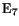Next we turn to the 133-dimensional exceptional Lie group. In 1954, Freudenthal  described this group as the automorphism group of a 56-dimensional octonionic structure now called a 'Freudenthal triple system'. We sketch this idea below, but first we give some magic square constructions. Vinberg's version of the magic square gives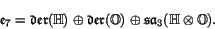Tits' version gives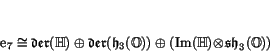and also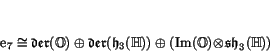The Barton-Sudbery version gives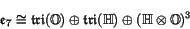Starting from equation (4) and using the fact that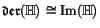is 3-dimensional, we obtain the elegant formula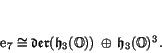This gives an illuminating way to compute the dimension of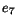: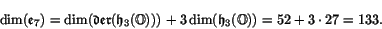Starting from equation (5) and using the concrete descriptions of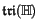and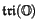from equation (3), we obtain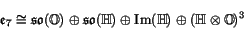Using equation (4.2), we may rewrite this as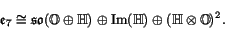Though not obvious from what we have done, the direct summandhere is really a Lie subalgebra of. In less octonionic language, this result can also be found in Adams' book :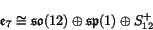He describes the bracket inin terms of natural operations involving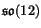and its spinor representation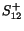. The funny-looking factor of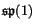comes from the fact that this representation is quaternionic. The bracket of an element ofand an element ofis the element ofdefined using the natural action ofon this space.

If we letbe the simply connected group with Lie algebra, it follows from results of Adams  that the subgroup generated by the Lie subalgebra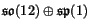is isomorphic to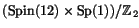. This lets us define the quateroctonionic projective plane by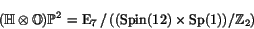and conclude that the tangent space at any point of this manifold is isomorphic to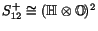. We can put an-invariant Riemannian metric on this manifold by the technique of averaging over the group action. It then turns out  that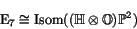and thus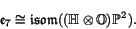Summarizing, we have the following 7 octonionic descriptions of:

Theorem 7   The compact real form ofis given by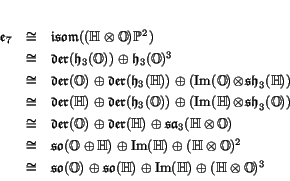where in each case the Lie bracket ofis built from natural bilinear operations on the summands.

Before the magic square was developed, Freudenthal  used another octonionic construction to study. The smallest nontrivial representation of this group is 56-dimensional. Freudenthal realized we can define a 56-dimensional space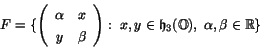and equip this space with a symplectic structure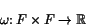and trilinear product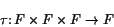such that the group of linear transformations preserving both these structures is a certain noncompact real form of, namely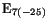. The symplectic structure and trilinear product onsatisfy some relations, and algebraists have made these into the definition of a 'Freudenthal triple system' [10,32,67]. The geometrical significance of this rather complicated sort of structure has recently been clarified by some physicists working on string theory. At the end of the previous section, we mentioned a relation between 9-dimensional Euclidean geometry and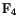, and a corresponding relation between 10-dimensional Lorentzian geometry and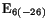. Murat Günaydin  has extended this to a relation between 10-dimensional conformal geometry and, and in work with Kilian Koepsell and Hermann Nikolai  has explicated how this is connected to Freudenthal triple systems.

Next: E8 Up: Exceptional Lie Algebras Previous: E6